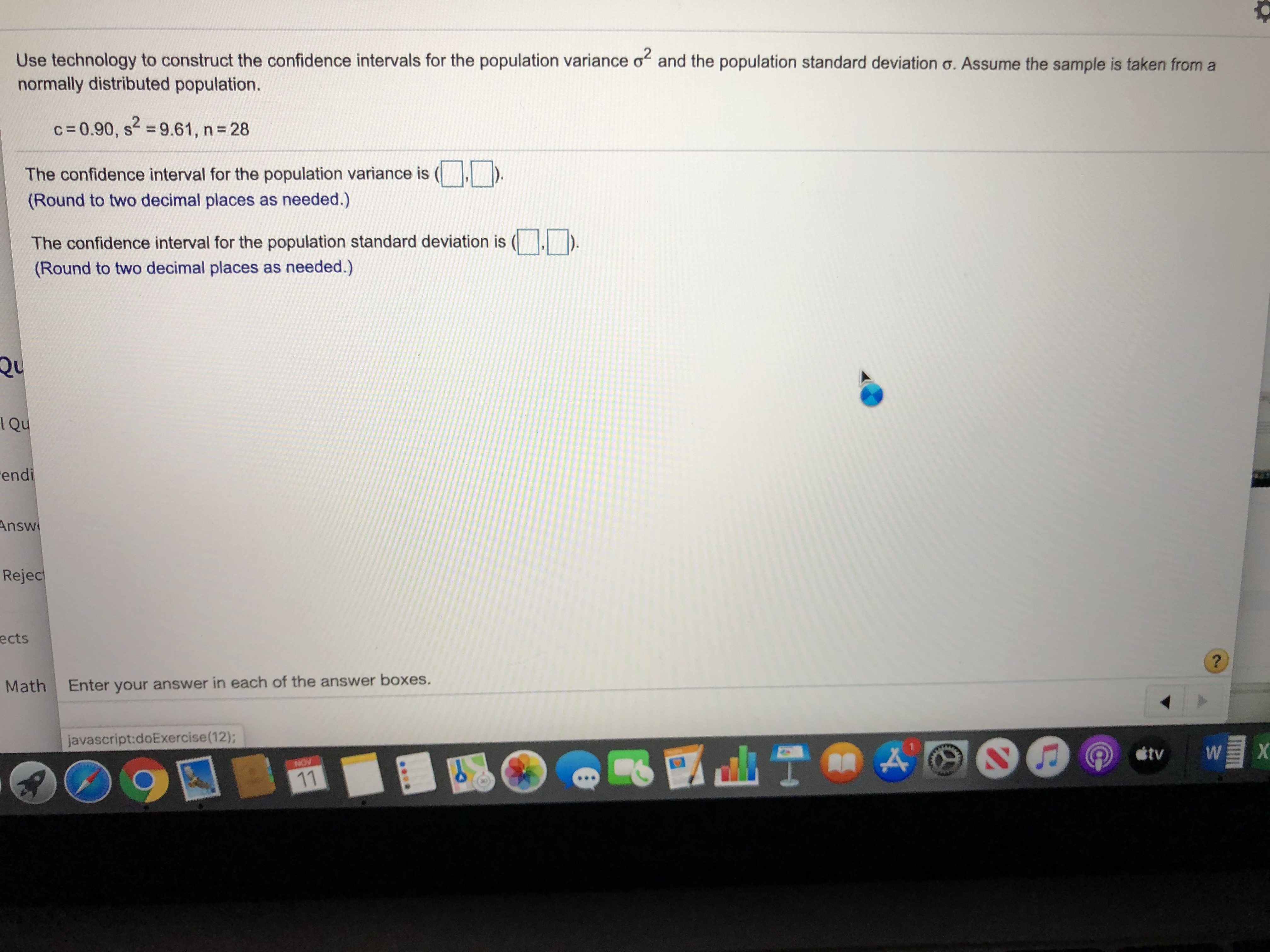# Use technology to construct the confidence intervals for the population variance o and the population standard deviation o. Assume the sample is taken from anormally distributed population.c 0.90, s 9.61, n 28The confidence interval for the population variance is(Round to two decimal places as needed.)|The confidence interval for the population standard deviation is(Round to two decimal places as needed.)QUQuendiAnswRejecects?MathEnter your answer in each of the answer boxes.javascript:doExercise(12);NOVtv110\$44090

Question
25 viewshelp_outlineImage TranscriptioncloseUse technology to construct the confidence intervals for the population variance o and the population standard deviation o. Assume the sample is taken from a normally distributed population. c 0.90, s 9.61, n 28 The confidence interval for the population variance is (Round to two decimal places as needed.) | The confidence interval for the population standard deviation is (Round to two decimal places as needed.) QU Qu endi Answ Rejec ects ? Math Enter your answer in each of the answer boxes. javascript:doExercise(12); NOV tv 11 0 \$4 4090 fullscreen
check_circle

Step 1

Given information:

Sample size (n) = 28

Confidence level = 0.90

Sample variance = 9.61

Step 2

Sample standard deviation is square root of sample variance. So,

Step 3

Significance level = 1- Confidence level

= 1- 0.90

= 0.10

Degree of...

### Want to see the full answer?

See Solution

#### Want to see this answer and more?

Solutions are written by subject experts who are available 24/7. Questions are typically answered within 1 hour.*

See Solution
*Response times may vary by subject and question.
Tagged in

### Measures